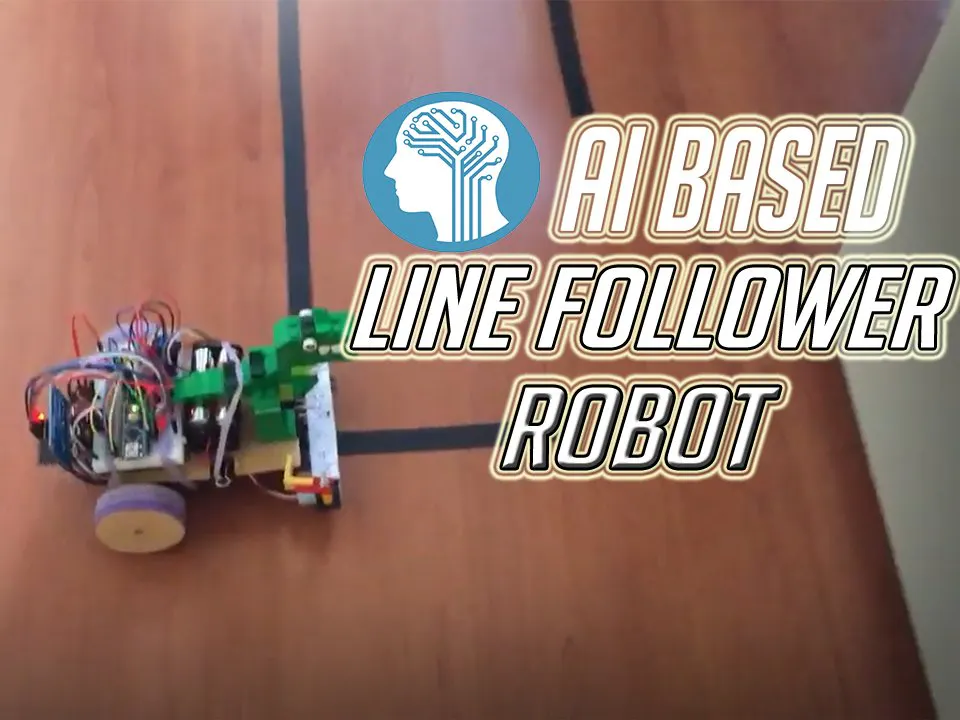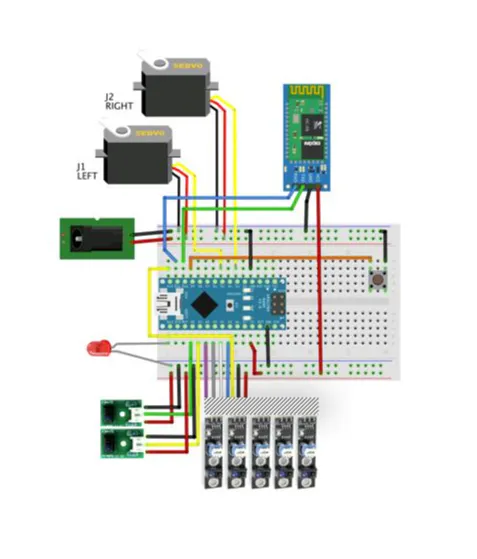# Artificial Intelligence (AI) Based Line Following Robot

Artificial Intelligence (AI) based line Following robot is a robot that a sense of detecting the correct path for its route.

IntermediateFull instructions provided10 hours11,036## Things used in this project

### Hardware components

 Arduino Nano
×1
 Seeed Studio Wireless Bluetooth RF Transceiver Master Slave
×1
 Amazon Web Services IR Infrared Sensor Module Reflective Photoelectric Light
×1
 Sony Continuous Rotation Servo
×1
 Android 4.8 Volt Battery
×1

### Software apps and online servicesArduino IDE

## Schematics

### Circuit Diagram## Code

### RoBot_Maze_Solve_2

C/C++
```/*------------------------------------------------------------------
7 sensors Smart Robot - Maze Solver and Line Follower with programable PID controller via BT
==> Basic movement based on Nano Mouse Robot, developed by Michael Backus (http://www.akrobotnerd.com/ )
==> Line follow based on http://samvrit.tk/tutorials/pid-control-arduino-line-follower-robot/?ckattempt=1
==> CREDIT to Patrick McCabe for the path Solving Code, visit patrickmccabemakes.com!!
Marcelo Jose Rovai - 23 April, 2016 - Visit: http://mjrobot.org
-------------------------------------------------------------------*/

#include <Servo.h>
#include "robotDefines.h"

String command;
String device;

// BT Module
#include <SoftwareSerial.h>
SoftwareSerial BT1(10, 11); // El pin 10 es Rx y el pin 11 es Tx

//---------------------------------------------
void setup()
{

Serial.begin(9600);
BT1.begin(9600);

pinMode(ledPin, OUTPUT);
pinMode(buttonPin, INPUT_PULLUP);

pinMode(lineFollowSensor0, INPUT);
pinMode(lineFollowSensor1, INPUT);
pinMode(lineFollowSensor2, INPUT);
pinMode(lineFollowSensor3, INPUT);
pinMode(lineFollowSensor4, INPUT);

// servos
leftServo.attach(5);
rightServo.attach(3);

BT1.print("check the PID constants to be sent to Robot");
Serial.print("check the PID constants to be sent to Robot");
BT1.println('\n');

{
checkBTcmd();  // verify if a comand is received from BT remote control
manualCmd ();
command = "";
}

checkPIDvalues();
mode = STOPPED;
status = 0; // 1st pass
}

void loop()
{
BT1.println("Start First Pass");
Serial.println("Start First Pass");
mazeSolve(); // First pass to solve the maze
BT1.println("End First Pass");
Serial.println("End First Pass");

{
checkBTcmd();  // verify if a comand is received from BT remote control
manualCmd ();
command = "";
}

BT1.println("Starting 2nd Pass");
Serial.println("Starting 2nd Pass");
pathIndex = 0;
status = 0;
mazeOptimization(); //run the maze as fast as possible
BT1.println("End 2nd Pass");
Serial.println("End 2nd Pass");

{
checkBTcmd();  // verify if a comand is received from BT remote control
manualCmd ();
command = "";
}

mode = STOPPED;
status = 0; // 1st pass
pathIndex = 0;
pathLength = 0;
}
```

### Functions

C/C++
```void mazeSolve(void)
{
while (!status)
{
switch (mode)
{
case NO_LINE:
motorStop();
goAndTurn (LEFT, 180);
recIntersection('B');
break;

case CONT_LINE:
runExtraInch();
if (mode != CONT_LINE) {goAndTurn (LEFT, 90); recIntersection('L');} // or it is a "T" or "Cross"). In both cases, goes to LEFT
else mazeEnd();
break;

case RIGHT_TURN:
runExtraInch();
if (mode == NO_LINE) {goAndTurn (RIGHT, 90); recIntersection('R');}
else recIntersection('S');
break;

case LEFT_TURN:
goAndTurn (LEFT, 90);
recIntersection('L');
break;

case FOLLOWING_LINE:
followingLine();
break;

}
}
}

//---------------------------------------------
void recIntersection(char direction)
{
path[pathLength] = direction; // Store the intersection in the path variable.
pathLength ++;
simplifyPath(); // Simplify the learned path.
}

//----------------------------------------------
void mazeEnd(void)
{
motorStop();
BT1.print("The End  ==> Path: ");
for(int i=0;i<pathLength;i++)
BT1.print(path[i]);
//Serial.print(path[i]);
BT1.println("");
Serial.print("  pathLenght ==> ");
Serial.println(pathLength);
status = 1;
mode = STOPPED;
}

//----------------------------------------------
void followingLine(void)
{
calculatePID();
motorPIDcontrol();
}

//------------------------------------------------------------------------------------------
// Path simplification.  The strategy is that whenever we encounter a
// sequence xBx, we can simplify it by cutting out the dead end.  For
// example, LBL -> S, because a single S bypasses the dead end
// represented by LBL.
void simplifyPath()
{
// only simplify the path if the second-to-last turn was a 'B'
if(pathLength < 3 || path[pathLength-2] != 'B')
return;

int totalAngle = 0;
int i;
for(i=1;i<=3;i++)
{
switch(path[pathLength-i])
{
case 'R':
totalAngle += 90;
break;
case 'L':
totalAngle += 270;
break;
case 'B':
totalAngle += 180;
break;
}
}

// Get the angle as a number between 0 and 360 degrees.
totalAngle = totalAngle % 360;

// Replace all of those turns with a single one.
switch(totalAngle)
{
case 0:
path[pathLength - 3] = 'S';
break;
case 90:
path[pathLength - 3] = 'R';
break;
case 180:
path[pathLength - 3] = 'B';
break;
case 270:
path[pathLength - 3] = 'L';
break;
}

// The path is now two steps shorter.
pathLength -= 2;

}

//----------------------------------------------------------------------------------------------
void mazeOptimization (void)
{
while (!status)
{
switch (mode)
{
case FOLLOWING_LINE:
followingLine();
break;
case CONT_LINE:
if (pathIndex >= pathLength) mazeEnd ();
else {mazeTurn (path[pathIndex]); pathIndex++;}
break;
case LEFT_TURN:
if (pathIndex >= pathLength) mazeEnd ();
else {mazeTurn (path[pathIndex]); pathIndex++;}
break;
case RIGHT_TURN:
if (pathIndex >= pathLength) mazeEnd ();
else {mazeTurn (path[pathIndex]); pathIndex++;}
break;
}
}
}

//-----------------------------------------------------
void mazeTurn (char dir)
{
switch(dir)
{
case 'L': // Turn Left
goAndTurn (LEFT, 90);
break;

case 'R': // Turn Right
goAndTurn (RIGHT, 90);
break;

case 'B': // Turn Back
goAndTurn (RIGHT, 800);
break;

case 'S': // Go Straight
runExtraInch();
break;
}
}
```

### GeneralFunctions.

C/C++
```void ledBlink(int times)
{
for (int i = 0; i<times; i++)
{
digitalWrite (ledPin, HIGH);
delay (500);
digitalWrite (ledPin, LOW);
delay (500);
}
}

//-----------------------------------------------------------------------------

void checkBTcmd()
{
while (BT1.available())   //Check if there is an available byte to read
{
delay(10); //Delay added to make thing stable
device += c; //build the string.
}
if (device.length() > 0)
{
Serial.print("Command received from BT ==> ");
Serial.println(device);
command = device;
device ="";  //Reset the variable
BT1.flush();
}
}

//------------------------------------------------------------------------
void manualCmd()
{
switch (command)
{
case 'g':
mode = FOLLOWING_LINE;
break;

case 's':
motorStop(); //turn off both motors
break;

case 'f':
motorForward();
break;

case 'r':
motorTurn(RIGHT, 30);
motorStop();

break;

case 'l':
motorTurn(LEFT, 30);
motorStop();
break;

case 'b':
motorBackward();
break;

case 'p':
Kp = command;
break;

case 'i':
Ki = command;
break;

case 'd':
Kd = command;
break;
}
}

//------------------------------------------------------------------------
void sendBTdata (int data) // send data to BT

{
digitalWrite (ledPin, HIGH);
BT1.print("Data from Arduino");
BT1.print(data);
BT1.print(" xx");
BT1.println('\n');
digitalWrite (ledPin, LOW);
}

//--------------------------------------------------------
void calculatePID()
{
P = error;
I = I + error;
D = error-previousError;
PIDvalue = (Kp*P) + (Ki*I) + (Kd*D);
previousError = error;
}

//--------------------------------------------------------
void checkPIDvalues()
{

BT1.print("PID: ");
BT1.print(Kp);
BT1.print(" - ");
BT1.print(Ki);
BT1.print(" - ");
BT1.println(Kd);

Serial.print("PID: ");
Serial.print(Kp);
Serial.print(" - ");
Serial.print(Ki);
Serial.print(" - ");
Serial.println(Kd);

}

//-----------------------------------------------
void testLineFollowSensors()
{

Serial.print ("LFS: L  0 1 2 3 4  R ==> ");
Serial.print (LFS0);
Serial.print (" ");
Serial.print (LFS1);
Serial.print (" ");
Serial.print (LFS2);
Serial.print (" ");
Serial.print (LFS3);
Serial.print (" ");
Serial.print (LFS4);
Serial.print ("  ==> ");

Serial.print (" P: ");
Serial.print (P);
Serial.print (" I: ");
Serial.print (I);
Serial.print (" D: ");
Serial.print (D);
Serial.print (" PID: ");
Serial.println (PIDvalue);
}
```

### sensorFuntions

C/C++
```//-------------------------------------------------------------

Sensor Array 	Error Value
0 0 0 0 1	 4
0 0 0 1 1	 3
0 0 0 1 0	 2
0 0 1 1 0	 1
0 0 1 0 0	 0
0 1 1 0 0	-1
0 1 0 0 0	-2
1 1 0 0 0	-3
1 0 0 0 0	-4

1 1 1 1 1        0 Robot found continuous line - test if an intersection or end of maze
0 0 0 0 0        0 Robot found no line: turn 180o

*/
{

if     ((LFSensor== 1 )&&(LFSensor== 1 )&&(LFSensor== 1 )&&(LFSensor== 1 )&&(LFSensor== 1 ))  {mode = CONT_LINE; error = 0;}
else if((LFSensor== 0 )&&                                                   (farRightSensor < THRESHOLD)) {mode = RIGHT_TURN; error = 0;}
else if ((farLeftSensor <  THRESHOLD)                                                  &&(LFSensor== 0 )) {mode = LEFT_TURN; error = 0;}
else if((LFSensor== 0 )&&(LFSensor== 0 )&&(LFSensor== 0 )&&(LFSensor== 0 )&&(LFSensor== 0 ))  {mode = NO_LINE; error = 0;}
else if((LFSensor== 0 )&&(LFSensor== 0 )&&(LFSensor== 0 )&&(LFSensor== 0 )&&(LFSensor== 1 ))  {mode = FOLLOWING_LINE; error = 4;}
else if((LFSensor== 0 )&&(LFSensor== 0 )&&(LFSensor== 0 )&&(LFSensor== 1 )&&(LFSensor== 1 ))  {mode = FOLLOWING_LINE; error = 3;}
else if((LFSensor== 0 )&&(LFSensor== 0 )&&(LFSensor== 0 )&&(LFSensor== 1 )&&(LFSensor== 0 ))  {mode = FOLLOWING_LINE; error = 2;}
else if((LFSensor== 0 )&&(LFSensor== 0 )&&(LFSensor== 1 )&&(LFSensor== 1 )&&(LFSensor== 0 ))  {mode = FOLLOWING_LINE; error = 1;}
else if((LFSensor== 0 )&&(LFSensor== 0 )&&(LFSensor== 1 )&&(LFSensor== 0 )&&(LFSensor== 0 ))  {mode = FOLLOWING_LINE; error = 0;}
else if((LFSensor== 0 )&&(LFSensor== 1 )&&(LFSensor== 1 )&&(LFSensor== 0 )&&(LFSensor== 0 ))  {mode = FOLLOWING_LINE; error =- 1;}
else if((LFSensor== 0 )&&(LFSensor== 1 )&&(LFSensor== 0 )&&(LFSensor== 0 )&&(LFSensor== 0 ))  {mode = FOLLOWING_LINE; error = -2;}
else if((LFSensor== 1 )&&(LFSensor== 1 )&&(LFSensor== 0 )&&(LFSensor== 0 )&&(LFSensor== 0 ))  {mode = FOLLOWING_LINE; error = -3;}
else if((LFSensor== 1 )&&(LFSensor== 0 )&&(LFSensor== 0 )&&(LFSensor== 0 )&&(LFSensor== 0 ))  {mode = FOLLOWING_LINE; error = -4;}

Serial.print (farLeftSensor);
Serial.print (" <== LEFT  RIGH==> ");
Serial.print (farRightSensor);
Serial.print ("  mode: ");
Serial.print (mode);
Serial.print ("  error:");
Serial.println (error);

}

//-----------------------------------------------------------

{

}
```

### RobotDefines.h

C/C++
```int mode = 0;

# define STOPPED 0
# define FOLLOWING_LINE 1
# define NO_LINE 2
# define CONT_LINE 3
# define POS_LINE 4
# define RIGHT_TURN 5
# define LEFT_TURN 6

const int power = 250;
const int iniMotorPower = 250;
int extraInch = 200;

const int ledPin = 13;
const int buttonPin = 9;

// LFSensor more to the Left is "0"
const int lineFollowSensor0 = 12;
const int lineFollowSensor1 = 18;
const int lineFollowSensor2 = 17;
const int lineFollowSensor3 = 16;
const int lineFollowSensor4 = 19;

const int farRightSensorPin = 0; //Analog Pin A0
const int farLeftSensorPin = 1; //Analog Pin A0
const int THRESHOLD = 150;
int farRightSensor = 0;
int farLeftSensor = 0;

int LFSensor={0, 0, 0, 0, 0};

// PID controller
float Kp=50;
float Ki=0;
float Kd=0;

float error=0, P=0, I=0, D=0, PIDvalue=0;
float previousError=0, previousI=0;

#define RIGHT 1
#define LEFT -1

Servo leftServo;
Servo rightServo;

//-------------------------------------------------
//Specific Maze Phase 2 (optimization) definitions and variables

unsigned char dir;

// The path variable will store the path that the robot has taken:
//  'L' for left
//  'R' for right
//  'S' for straight (going straight through an intersection)
//  'B' for back (U-turn)

char path = "";
unsigned char pathLength = 0; // the length of the path
int pathIndex = 0;
unsigned int status = 0; // solving = 0; reach end = 1
```

### MotorFuntions

C/C++
```void motorStop()
{
leftServo.writeMicroseconds(1500);
rightServo.writeMicroseconds(1500);
delay(200);
}

//---------------------------------------------
void motorForward()
{
rightServo.writeMicroseconds(1500 + power);
}

//---------------------------------------------
void motorBackward()
{
leftServo.writeMicroseconds(1500 + power);
rightServo.writeMicroseconds(1500 - power);
}

//---------------------------------------------
void motorFwTime (unsigned int time)
{
motorForward();
delay (time);
motorStop();
}

//---------------------------------------------
void motorBwTime (unsigned int time)
{
motorBackward();
delay (time);
motorStop();
}

//------------------------------------------------
void motorTurn(int direction, int degrees)
{
rightServo.writeMicroseconds(1500 - iniMotorPower*direction);
motorStop();
}

//---------------------------------------------------
void motorPIDcontrol()
{

int leftMotorSpeed = 1500 - (iniMotorPower+adj) - PIDvalue;
int rightMotorSpeed = 1500 + iniMotorPower - PIDvalue;

// The motor speed should not exceed the max PWM value
constrain(leftMotorSpeed, 1000, 2000);
constrain(rightMotorSpeed, 1000, 2000);

leftServo.writeMicroseconds(leftMotorSpeed);
rightServo.writeMicroseconds(rightMotorSpeed);

//Serial.print (PIDvalue);
//Serial.print (" ==> Left, Right:  ");
//Serial.print (leftMotorSpeed);
//Serial.print ("   ");
//Serial.println (rightMotorSpeed);
}

//---------------------------------------------------
void runExtraInch(void)
{
motorPIDcontrol();
delay(extraInch);
motorStop();
}

//---------------------------------------------------
void goAndTurn(int direction, int degrees)
{
motorPIDcontrol();